Different ways of expressing Boolean Functions

Consider the Boolean Equation: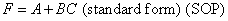Its Truth Table is: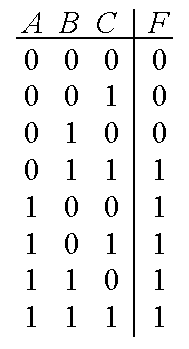The Rules of Minterms and Maxterms are shown in this table:A Minterm is valid where the function is equal to ONE.... and a Maxterm is valid where the function is equal to ZERO

So applying this to our function gives Minterm expression: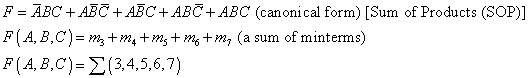Minterms must be combined using the OR operator... so the expression is called Sum of Products

and Maxterm expression: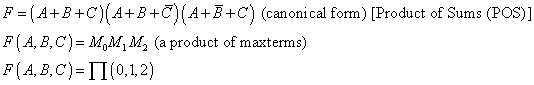Maxterms must be combined using the AND operator... so the expression is called Product of Sums

A "CANONICAL" expression contains ALL the literals (variables) in EVERY term.
A "STANDARD" expression is an SOP or POS expression but does not have to contain all literals in every term.
A "NON-STANDARD" expression is one that contains MIXED operators within parentheses for example: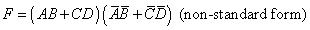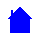ELET1210 Homepage